# Sinc Interpolation Examples

### Matlab code for sinc interpolation

```function y = sinc_interp(x,u)

m = 0:length(x)-1;

for i=1:length(u)
y(i) = sum(x.*sinc(m- u(i)));
end
```

## Example 1

The function is the sequence x[n] = n a^n u[n].

```a = 0.9;
N = 64;
n = 0:N-1;
x = n.*a.^n;
```

### Sampled values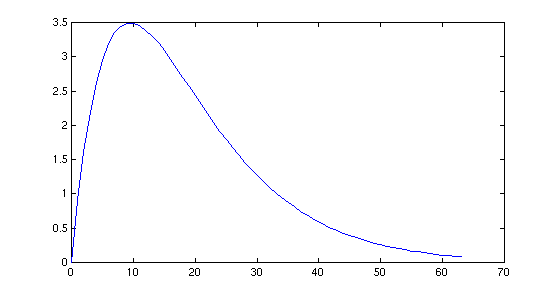```plot(n,x);
```

### Magnitude of Fourier transform

The function is essentially band-limited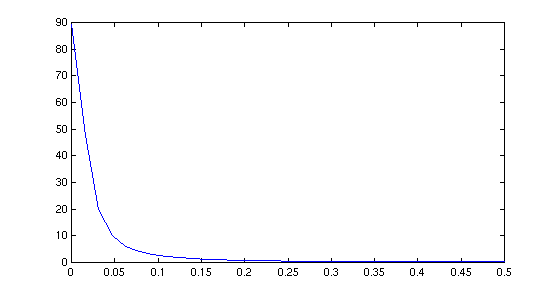```y = fft(x);
k= 0:N/2;
plot(k/N,abs(y(1:N/2+1)));
```

### Interpolations

The black curve is the Matlab `interp` method. The blue curve is sinc interpolation. The circles are sample values.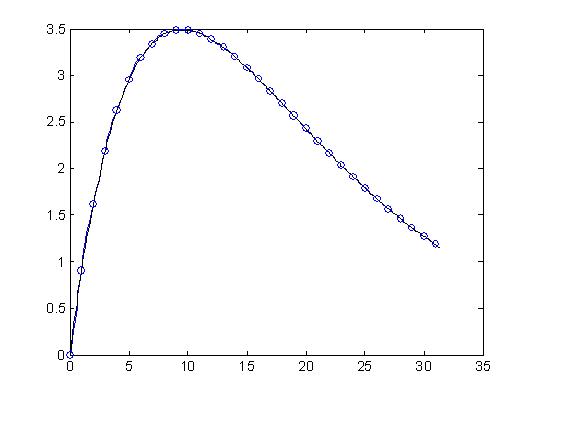```s = linspace(0,63,512);
x2 = sinc_interp(x,s);
plot(s(1:256),x2(1:256));
hold
xi = interp1(n,x,s);
plot(s(1:256),xi(1:256),'k');
plot(n(1:N/2),x(1:N/2),'o');
hold off
```

The first half of the data is shown.

### Interpolation difference

The following shows the difference between the two interpolation methods.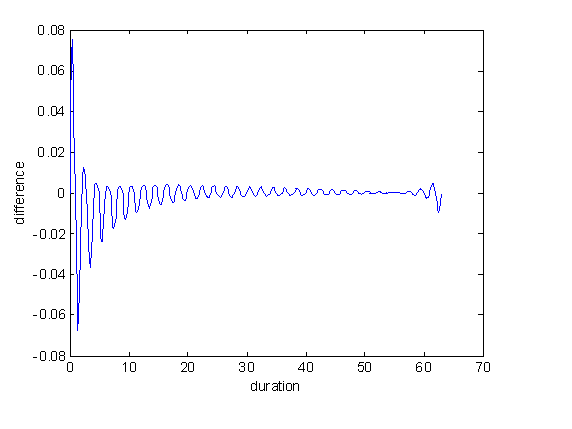```plot(s,xi-x2);
ylabel('difference');
xlabel('duration');
```

## Example 2

The function is the sequence x[n] = a^n u[n].

```a = 0.9;
N = 64;
n = 0:N-1;
x = a.^n;
```

### Sampled values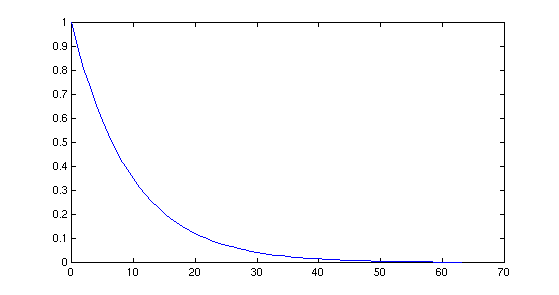### Magnitude of Fourier transform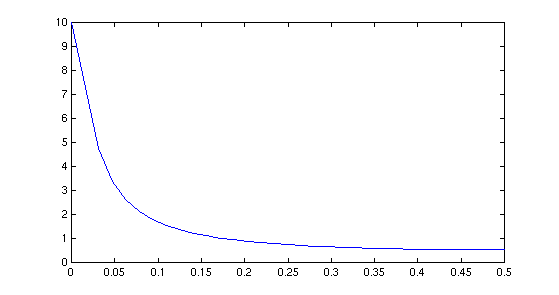Not exactly band-limited

### Interpolations

The black curve is the Matlab `interp` method. The blue curve is sinc interpolation. The circles are sample values.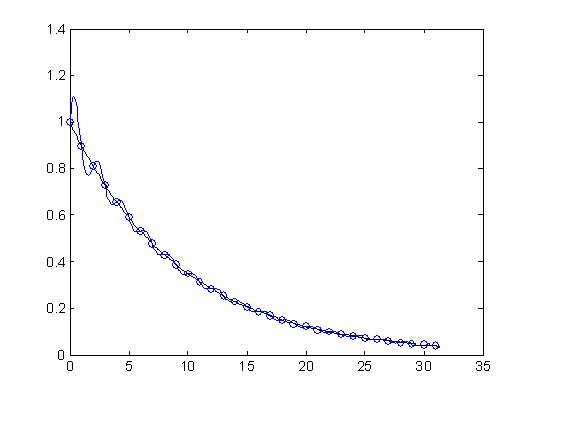### Interpolation difference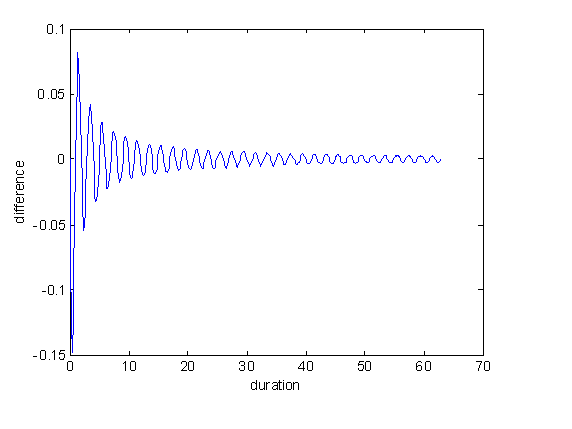## Conclusion

sinc interpolation is seldom optimal. It almost always exhibits ringing, sometimes severely.

Maintained by John Loomis, last updated 26 Oct 2005